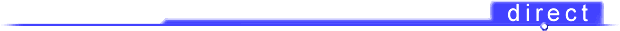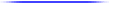to the 16th Edition IEE Regulationschapter 1 The IEE Regulations chapter 2 Installation Requirements and Characteristics chapter 3 Installation Control and Protection chapter 4 Cables, Conduits and Trunking chapter 5 Earthing chapter 6 Circuits chapter 7 Special Installations chapter 8 Testing and Inspection chapter 9 Data cabling and NetworksEarthing
 5.1 - The earthing principle 5.6 - Protective multiple earthing (PME) 5.2 - Earthing Systems 5.7 - Earthed concentric wiring 5.3 - Earth fault loop impedance 5.8 - Other protection methods 5.4 - Protective conductors 5.9 - Residual current devices (RCDs) 5.10 - Combined functional and protective ---------earthing
 5.3.1 - Principle 5.3.2 - The importance of loop impedance 5.3.3 - The resistance/impedance relationship 5.3.4 - Earth-fault loop impedance values 5.3.5 - Protective conductor impedance 5.3.6 - Maximum circuit conductor length

5.3.6 - Maximum circuit conductor length

The complete earth-fault loop path is made up of a large number of parts as shown in {Fig 5.7}, many of which are external to the installation and outside the control of the installer. These external parts make up the external loop impedance (Ze). The rest of the earth-fault loop impedance of the installation consists of the impedance of the phase and protective conductors from the intake position to the point at which the loop impedance is required.

Since an earth fault may occur at the point farthest from the intake position, where the impedance of the circuit conductors will be at their highest value, this is the point which must be considered when measuring or calculating the earth-fault loop impedance for the installation. Measurement of the impedance will he considered in {8.6.2}. Provided that the external fault loop impedance value for the installation is known, total impedance can be calculated by adding the external

 Table 5.5 - Resistance per metre of copper conductors at 20°C for ---------------- calculation of R1 + R2 Conductor cross-sectional area (mm²) Resistance per metre run (m ohms / m) 1.0 18.1 1.5 12.10 2.5 7.41 4.0 4.61 6.0 3.08 10.0 1.83 16.0 1.15 25.0 0.727 Note that to allow for the increase in resistance with increased temperature under fault conditions the values of {Table 5.5} must be multiplied by 1.2 for p.v.c. insulated cables (see {Table 8.7})

impedance to that of the installation conductors to the point concerned. The combined resistance of the phase and protective conductors is known as R1+ R2. The same term is sometimes used for the combined resistance of neutral and protective conductors (see {8.4.1}). In the vast majority of cases phase and neutral conductors have the same cross-sectional area and hence the same resistance.

For the majority of installations, these conductors will be too small for their reactance to have any effect (below 25 mm² cross-sectional area reactance is very small), so only their resistance's will be of importance. This can be measured by the method indicated in {Fig 5.9}, remembering that this time we are interested in the combined resistance of phase and protective conductors, or can be calculated if we measure the cable length and can find data concerning the resistance of various standard cables. These data are given here as {Table 5.5}.

The resistance values given in {Table 5.5} are for conductors at 20°C. Under fault conditions the high fault current will cause the temperature of the conductors to rise and result in an increase in resistance. To allow for this changed resistance, the values in {Table 5.5} must be multiplied by the appropriate correction factor from Table {8.7}. It should be mentioned that the practice which has been adopted here of adding impedance and resistance values arithmetically is not strictly correct. Phasor addition is the only perfectly correct method since the phase angle associated with resistance is likely to he different from those associated with impedance, and in addition impedance phase angles will differ from one another. However, if the phase angles are similar, and this will be so in the vast majority of cases where electrical installations are concerned, the error will be acceptably small.

It is often assumed that higher conductor temperatures are associated with the higher levels of fault current. In most cases this is untrue. A lower fault level will result in a longer period of time before the protective device operates to clear it, and this often results in higher conductor temperature.

Example 5.1
A 7 kW shower heater is to be installed in a house fed with a 240 V TN-S supply system with an external loop impedance (Ze) of 0.8 Ohms. The heater is to be fed from a 32 A BS 88 cartridge fuse. Calculate a suitable size for the p.v.c. insulated and sheathed cable to be used and determine the maximum possible length of run for this cable. It may be assumed that the cable will not be subject to any correction factors and is clipped direct to a heat conducting surface throughout its run.

 First calculate the circuit current. I = P = 7000 A = 29.2 A U 240

Next, select the cable size from {Table 4.7} (which is based on [Table 4D2A]) from which we can see that 4 mm² cable of this kind clipped direct has a rating of 36 A. The 2.5 mm² rating of 27 A is not large enough. We shall assume that a 2.5 mm² protective conductor is included within the sheath of the cable.

Now we must find the maximum acceptable earth-fault loop impedance for the circuit. Since this is a shower, a maximum disconnection time of 0.4 s will apply, so we need to consult {Table 5.1}, which gives a maximum loop impedance of 1.09 Ohms for this situation. Since the external loop impedance is 0.8 Ohms, we can calculate the maximum resistance of the cable.

 R cable = max. loop impedance - external impedance =1.09 - 0.8 Ohms = 0.29 Ohms

This assumes that resistance and impedance phase angles are identical, which is not strictly the case. However, the difference is unimportant.

The phase conductor (4 mm²) has a resistance of 4.6 m Ohms/m and the 2.5 mm² protective conductor 7.4 mOhms/m, so the combined resistance per metre is 4.6 + 7.4 = 12.0 m Ohms/m. The cable will get hot under fault conditions, so we must apply the multiplier of 1.2.

 Effective resistance of cable per metre = 12.0 x 1.2 m Ohms/m = 14.4 m Ohms/m

The maximum length of run in metres is thus the number of times 14.4 mOhms will divide into 0.29 Ohms.

 Maximum length of run = 0.29 x 1000 m = 20.1 m 14.4

This is not quite the end of our calculation, because we must check that this length of run will not result in an excessive volt drop. From {Table 4.7} based on [Table 4D2B] a 4 mm² cable of this kind gives a volt drop of 11 mV/A/m.

 Volt drop = 11 x circuit current (A) x length of run (m) divided by 1000 Volt drop = 11 x 29.2 x 20.1 V = 6.46 V 1000

Since the permissible volt drop is 4% of 240 V or 9.6 V, this length of run is acceptable.